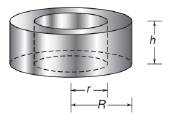Chapter 9.3, Problem 34E### Elementary Geometry for College St...

6th Edition
Daniel C. Alexander + 1 other
ISBN: 9781285195698

#### Solutions

Chapter
Section### Elementary Geometry for College St...

6th Edition
Daniel C. Alexander + 1 other
ISBN: 9781285195698
Textbook Problem
1 views

# In Exercises 34 and 35, give a paragraph proof for each claim.The total area T of a right circular cylinder whose altitude is of length h and whose circular bas has a radius of length r is given by T = 2 π r ( r + h ) .To determine

To give:

The paragraph proof for the claim “The total area T of a right circular cylinder whose altitude is of length h and whose circular base has a radius of length r is given by T=2πr(r+h)”.

Explanation

Given:

The claim is “The total area T of a right circular cylinder whose altitude is of length h and whose circular base has a radius of length r is given by T=2πr(r+h)”.

Proof:

Consider a right circular cylinder.

Let r be the radius of the base of the cylinder and h be the altitude of the cylinder.

The lateral area of the right circular cylinder is L=2πrh.

The base of the cylinder is a circle with radius r.

The base area of the right circular cylinder is B=πr2.

Hence the total area of the right circular cylinder is T=L+B

### Still sussing out bartleby?

Check out a sample textbook solution.

See a sample solution

#### The Solution to Your Study Problems

Bartleby provides explanations to thousands of textbook problems written by our experts, many with advanced degrees!

Get Started